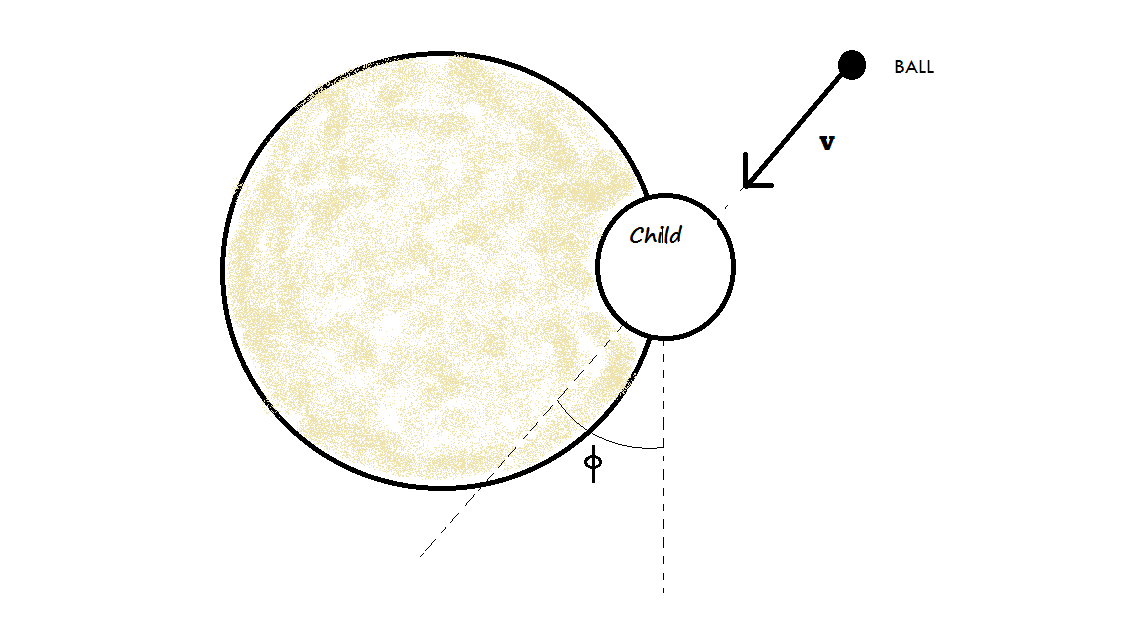# Two boys at the merry go round!In Figure above, a $30 kg$ child stands on the edge of a stationary merry-go-round of radius $2.0 m$. The rotational inertia of the merry-go-round about its rotation axis is $150 kg m^{2}$• The child catches a ball of mass $1.0 kg$ thrown by a friend. Just before the ball is caught, it has a horizontal velocity $\vec{v}$ of magnitude $12 m/s$, at angle $\phi= 37°$ with. a line tangent to the outer edge of the merry-go-round, as shown. What is the angular speed (in rad/sec) of the merry-go-round just after the ball is caught?

Liked it try some more

×